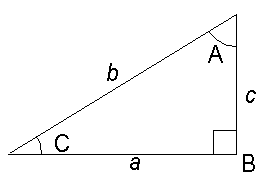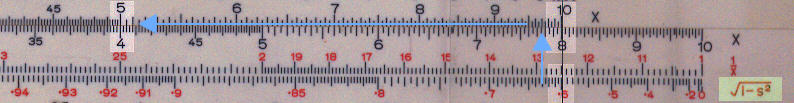Pythagorean

This applies to rules with a P scale.

To find the value of Ö(1-x2):

• Move the cursor to the x value on the D scale.
• Read the value of Ö(1-x2) on the P scale.

This scale can be used either for right angled triangles or for converting sin to cos (and vice versa).

For right angled triangles, c2 = b2 - a2then
c = b Ö(1- (a/b)2 )

Example:
b=5.0 and a = 3.0 How long is c
Calculate a/b = 3.0/5.0 = 0.6
Cursor to 0.6 of Pythagorean scale (marked Ö(1-s2) and highlighted in green.)
10 on C to cursor,
Answer (4.0) under 5.0 on D.For cosine the formula is:

cos = Ö(1- sin2)